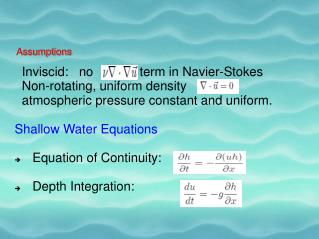DownloadDownload PresentationAssumptions

# Assumptions

Download Presentation## Assumptions

- - - - - - - - - - - - - - - - - - - - - - - - - - - E N D - - - - - - - - - - - - - - - - - - - - - - - - - - -
##### Presentation Transcript

1. Inviscid: no term in Navier-Stokes Non-rotating, uniform density atmospheric pressure constant and uniform. Shallow Water Equations Equation of Continuity: Depth Integration: Assumptions

2. Equation of Continuity m = density( ) * h* dx Dx = u*dt m = *h*u*dt

3. Pressure increases with depth according to overhead mass per unit area. Pressure at depth h-z: Integrating Hydrostatic Balance

4. But, => Therefore Net Force => F = Fs + F1 – F2 Thus we get, Depth Integration

5. => (h)tx= - (uh)xx => (u)tt = - g(h)tx Eliminating (h)tx on both sides, (uh) xx - 1/g*u tt = 0. (u)xx – (1/gh)*utt=0 (Hyperbolic PDE) Wave Equation -> c2(u)xx – utt = 0 Thus, c=root(gh); - Tushar Athawale. Shallow Water Equation